# Performance of Photovoltaic Thermal Technology Using V-Absorber with Exergy, Improvement Potential and Sustainability Index Analysis

Performance of Photovoltaic Thermal Technology Using V-Absorber with Exergy, Improvement Potential and Sustainability Index Analysis
Department of Physics Education, Universitas Islam Negeri Mataram, Mataram 83116, Indonesia

Department of Information, Sumbawa University of Technology, Sumbawa Besar 84371, Indonesia

Department of Mathematics Education, Universitas Islam Negeri Mataram, Mataram 83116, Indonesia

Department of Information Systems and Technology, Universitas Muhammadiyah Mataram, Mataram 83113, Indonesia

Corresponding Author Email:
zohri.ukm@gmail.com
Page:
551-557
|
DOI:
https://doi.org/10.18280/mmep.100223
17 January 2023
|
Revised:
5 March 2023
|
Accepted:
12 March 2023
|
Available online:
28 April 2023
| Citation

OPEN ACCESS

Abstract:

This research aims to test the PVT system's performance using V-Absorber model. The PVT technology using V-Absorber is conducted using a mathematical approach. This mathematical or theoretical approach aims to analyse suitable variables in predicting the system's optimal performance. The analysis of this system is the analysis of exergy and the most important is the analysis of the sustainability index and improvement potential. The variable values of sunlight intensity are 385 W/m2, 575 W/m2 and 815 W/m2. At the same time, the flow air is from 0.01 kg/s to 0.10 kg/s. The analysis results show that the position of the flow air of 0.06 kg/s is the maximum value for exergy using V-Absorber. The optimum sustainability index's value was 2.012 at flow air of 0.06 kg/s with sunlight intensity of 815 W/m2. For the improvement potential, the optimum yield was 329.24 Watt for flow air of 0.10 kg/s. The results of the sustainability index and improvement potential analysis show that the effect of the flow air and sunlight intensity was very significant.

Keywords:

PVT technology, V-Absorber, exergy analysis, sustainability index, improvement potential

1. Introduction

The use of fossil fuels to generate electricity is estimated at around 90%. The electricity obtained from fossil fuels is processed through combustion. The combustion process will release smoke or particles that can provide pollutants or pollution to the air and atmosphere. Fossil fuel combustion also releases large amounts of carbon dioxide into the air. Increased carbon dioxide can cause the greenhouse effect and cause climate change . Air pollution is a grave problem for humans. The release of carbon dioxide and smoke is caused by burning fossil fuels. Thick smoke will endanger the health of humans, animals, and plants on earth. Therefore, efforts to reduce greenhouse gases should continue maximally by optimizing the future use of new and renewable energy .

Energy sources from fossil fuels are energy sources that produce significant environmental impacts. On the other hand, energy sources from fossil fuels continue to decrease or deplete. This situation encourages researchers around the globe to develop new and renewable energy . The nature of new and renewable energy is clean energy and future energy. One of the renewable energies is solar energy which can be obtained anywhere as long as the sun still shines . Solar energy can be converted into electrical energy and heat energy. One technology that can produce electrical energy from the sun is photovoltaic . Specifically, Photovoltaic Thermal technology can produce electrical and thermal energy simultaneously .

Coolant technology in solar panels or photovoltaics has three kinds of cooling. These three types are active, passive cooling, and a combination of passive active. One can integrate coolant technology in solar panels with other new technologies . Active cooling technologies typically use an air pump or fan to drive cooling air, using water media and nanofluidic media . In addition to active air coolant media, passive cooling media are widely used, including authentic coolers, cotton wicks, paraffin wax, organic materials, et cetera. Active coolant media requires power or energy to work . At the same time, passive coolant media does not require energy to work . PVT technology with flat plate collector coolers has evolved over the last few years. Reviews of plate collector coolant technology have been discussed since before 2010. One may find it difficult to get a PVT system with a flat plate collector in the market . Many reviews identified plate collectors from the perspective of financing, certification, efficiency integration, et cetera. However, this review aims to enhance the market development of PVT flat plate collector technology .

The application of PVT system technology has been widely applied in various technologies, saving electricity and heat energy. PVT system using water fluids and air as the coolant medium has been widely tested . The combination of various kinds of air and water collectors or a combination of air and water collectors to cool the photovoltaic so that the efficiency level is preserved [14, 15]. The performance of a system or process can be assessed through its outcome effectively using exergy analysis. The ratio between the output and input values in a system operating at maximum state is called the second law of thermodynamics . The concept of exergy is the one that can assess system performance and as a consideration in designing, evaluating, analyzing, and improving the performance of a PVT system [17, 18].

Fudholi et al.  has conducted cost analysis on a collector using fins and without fins in drying application. The exergy analysis results of the fins collector show 6% to 30% values. Fudholi et al.  also study the fins application in the Improvement potential approach in solar collector technology. The result of achieved improvement potential ranges from 740 W-1070 W. The analysis of the exergy approach is widely used in solar collector applications or photovoltaic thermal (PVT) technology because the exergy approach greatly determines the quality of a tested system . Benli  made several shapes of solar collector designs, such as trapezium shape, plate collector shape, and inverted trapezium shape. After analyzing the results using the exergy approach, he recommended a new design to analyze the exergy and energy values of the system. Meanwhile, Hedayatizadeh et al.  designed a solar collector using the ∇-groove model. The design of the ∇-groove collector is studied using the effect of temperature changes on the collector when the air produced in the collector enters or exits.

PVT technology employing nanofluid has been studied using an exergy and energy analysis approach. The result of exergy efficiency is 15%, while the energy analysis is 13.2%. This result implies that the effect of nanofluid coolant is somewhat significant [24, 25]. Salem et al.  has made aluminum coolant plates application on PVT technology. This cooling plate is made in a straight channel and a helical model shape. The exergy efficiency value generated starts from 11.1%-13.4%. At the same time, the energy efficiency value ranges from 59.3% to 92%. These energy and exergy results indicate an increase in employing aluminum cooling plates. Exergoeconomic and environmental analysis approaches are still rarely studied in PVT systems. Tripathi et al.  analyzed the PVT system using exergoeconomic and environmental analysis on PVT technology with a condenser collector.

Exergoeconomic and Enviroeconomic analyses of solar distillation systems combined with PVT technology were reported by Tiwari et al. . The study was conducted in New Delhi, employing an experimental approach. PVT's thermal and total energy efficiencies are 13.4%-23.2% and 28.5-53.4%, respectively. Jahromi et al.  conducted energy, exergy, and economic analyses. The research was conducted on real climatic conditions in Iran. This PVT system's exergy efficiency is 9.6%-9.7%. The use of semi-transparent solar panels in PVT Technology has been designed by Rajoria et al. . The analysis used is energy, exergy, and enviroeconomic analysis. Such result of the electrical energy efficiency is 3.1-9.1%, while the thermal energy efficiency is 12.1-28.1%. Transparent model solar panels began to be used around 2013. Kamthania and Tiwari  designed a hybrid semi-transparent PVT technology using various silicon and non-silicon. The semi-transparent PV efficiency is 12%.

Collector design using V-Absorber is a good design for the solar collector. Implementing a V-Absorber to generate air heat on a solar collector system is a good one to enhance energy and exergy yield in the solar collector system or PVT technology. The use of energy usefully for PVT technology is a form of exergy. The exergy method is a very effective approach for testing and optimizing thermodynamic systems in PVT technology. by getting the exergy and exergy efficiency values, we will be able to calculate the Sustainability Index assessment (SI) and improvement potential value in PVT technology.

Analysis of the V-Absorber model's sustainability index and improvement potential approach in PVT systems is rarely used. Because the sustainability index and improvement potential approach is excellent for ensuring the sustainability of a PVT system in the future, this mathematical model approach is expected to predict parameters or variables suitable for PVT technology with V-Absorber. The purpose of this study was to analyse the sustainability index, improvement potential and exergy on PVT technology systems with V-Absorber by a theoretical approach or mathematical modelling.

2. Method

The use of a V-Absorber is to cool the PV panel from overheating conditions in the PVT technology . the design of the V-Absorber increased the electrical and thermal performance . In this study, a V-Absorber was designed with a height of 50 mm and a triangle of 60o angles for these edges as shown in Figure 1. Employing the law of energy balance, a heat transfer equation can be made by referring to the incoming energy equal to the outgoing energy in the PVT system using the V-Absorber model  as shown in Table 1.

## 11.pngFigure 1. Heat transfer for PVT technology with V-Absorber

Table 1. Parameters of the heat transfer of V-Absorber

 Parameters Equations For PV Panel $\alpha_c I\left(1-\eta_c\right)=U_t\left(T_c-T_a\right)+h_{c f 1}\left(T_c-T_{f 1}\right)-h_{r, v c}\left(T_v-T_c\right)$ (1) For the first channel of flow $2 \dot{m} C\left(T_{f 1, o}-T_{f 1, i}\right) / W L=h_{c f 1}\left(T_c-h_{f 1}\right)+h_{v f 1}\left(T_v-T_{f 1}\right)$ (2) For the V-Absorber $\alpha_v \tau_c I\left(1-\eta_c\right)=h_{r, v c}\left(T_v-T_c\right)+h_{v f 1}\left(T_v-T_{f 1}\right)+h_{r, v b}\left(T_v-T_b\right)+h_{v f 2}\left(T_v-T_{f 2}\right)$ (3) For the second channel of flow $2 \dot{m} C\left(T_{f 2 o}-T_{f 2, i}\right) / W L=h_{v f 2}\left(T_v-T_{f 2}\right)+h_{b f 2}\left(T_b-T_{f 2}\right)$ (4) For the bottom $h_{r, v b}\left(T_v-T_b\right)=h_{b f 2}\left(T_b-T_{f 2}\right)+U_b\left(T_b-T_a\right)$ (5) Matrix 5x5 from equation 1 to 5 $\left[\begin{array}{ccccc}B_1 & -h_{c f 1} & -h_{r p c} & 0 & 0 \\ h_{c f 1} & B_3 & h_{v f 2} & 0 & 0 \\ -h_{r p c} & -h_{v f 2} & B_5 & B_6 & -h_{r p b} \\ 0 & B_8 & B_9 & B_{10} & h_{b f 2} \\ 0 & 0 & h_{r p b} & h_{b f 2} & B_{12}\end{array}\right]\left[\begin{array}{c}T_c \\ T_{f 1} \\ T_v \\ T_{f 2} \\ T_b\end{array}\right]=\left[\begin{array}{c}B_2 \\ B_4 \\ B_7 \\ B_{11} \\ B_{13}\end{array}\right]$ The variables in matrix $B_1=U_t+h_{r p c}+h_{c f 1}$ $B_2=U_t T_a+\alpha_c I\left(1-\eta_c\right)$ $B_3=-\left[h_{c f 1}+h_{v f 1}+\left(\frac{2 \dot{m} C}{W L}\right)\right]$ $B_4=-\left(\frac{2 \dot{m} C}{W L}\right) T_i$ $B_5=h_{v f 1}+h_{v f 2}+h_{r p c}+h_{r p b}$ $B_6=-h_{v f 2}$ $B_7=\alpha_p \tau_c I\left(1-\eta_c\right)$ $B_8=4 \dot{m} C / W L$ $B_9=-B_6$ $B_{10}=-\left[h_{v f 2}+h_{b f 2}+\left(\frac{2 \dot{m} C}{W L}\right)\right]$ $B_{11}=-B_4$ $B_{12}=-\left(h_{b f 2}+h_{r p b}+U_b\right)$ $B_{13}=-T_a U_b$

From Eqns. (1) to (5), we estimate the temperature vector by inversion the matrix in excel software.

$[T]=[A]^{-1}[C]$

The normal matrix inversion subprogram is named to invert [A]-1 to calculate a new set of temperature matrices [T']. Initial estimation temperature [T] is compared with new temperature [T'] until the temperature value is converged. The difference between the initial and new temperature values reaches 0.001°C. In that case, matrix repetition is stopped, and the old temperature is substituted by a new one which is calculated and considered the required temperature for the part concerned. This process achieved appropriate convergence for the temperature of photovoltaic (Tp), the temperature of air (Tf), and the temperature of insulation (Tb). The iteration process is from 3 to 4 times. Subsequently, the PVT system's exergy value and sustainability index were calculated using the V-Absorber model as in Table 2.

Table 2. Parameter and equation for exergy, sustainability index and improvement potential

 Parameters Equations Destruction Exergy  $\sum E x_d=\sum E x_i-\sum\left(E x_{t h}-E x_p\right)$ Input of exergy $E x_i=A N I\left[1-\frac{4}{3}\left(\frac{T_a}{T_s}+\frac{1}{3}\left(\frac{T_a}{T_s}\right)^4\right)\right]$ Output of exergy $\sum\left(E x_{t h}-E x_p\right)=\sum E x_i-\sum E x_d$ thermal energy of PVT technology $E x_{t h}=\dot{m} C\left(T_o-T_i\right)\left[1-\frac{T_a+273}{T_o+273}\right]$ Exergy of PV technology $E x_p=\eta_p A S$ The exergy of the PVT technology $E x_{p v t}=E x_{t h e r m a l}+E x_{p v}$ Exergy efficiency $\eta_{\text {exergy }}=\frac{E x_{\text {output }}}{E x_{\text {input }}}$ Sustainability Index (SI) [4-18] $S I=\frac{1}{1-\eta_{\text {exergy }}}$ Improvement potential (IP) $I P=\left(1-\eta_{\text {exergy }}\right) E_{x e r g y_{\text {destruction }}}$ For efficiency of PV technology $\eta_{p v}=\eta_{r e f}\left[1-\beta_{r e f}\left(T_{p v}-T_{r e f}\right)\right]$ For the coefficient of temperature $\beta_{r e f}=\frac{1}{\left(T_o-T_{r e f}\right)}$ For efficiency of thermal $\eta_{t h}=\dot{m} C\left(T_o-T_i\right) / I A$ For Specific heat of air $C=1.0057+0.000066(T-27)$ For density of air $\rho=1.1774-0.00359(T-27)$ For conductivity of Thermal $k=0.02624+0.0000758(T-27)$ For Viscosity $\mu=[1.983+0.00184(T-27)] 10^{-5}$ For heat transfer coefficient of wind $h_w=2.8+3.3 \mathrm{~V}$ For Temperature of sky $T_s=0.0552 T_a^{1.5}$ For heat transfer coefficient of V-Absorber $h=3 k N u / 4 e$ For Nusselt number $N u=N u_o+\beta \frac{b}{L} n$ For laminar flow of air (Re<2800) $N u_o=2.281, \beta=0.126 R e$ For transition flow of air (2800≤Re≤104): $N u_o=1.9 \times 10^{-6} R e^{1.79}, \beta=225$ For turbulent flow of air (104
3. Results and Discussion

Figures 2, 3 and 4 demonstrate the comparison between flow of air versus input exergy, output exergy and destruction of exergy using V-Absorber. The variation of flow air is from 0.01 kg/m to 0.10 kg/m, and the sunlight intensity is 385 W/m2, 575 W/m2 and 815 W/m2. This study used a theoretical approach or mathematical modelling. The mathematical calculations show that the optimum exergy output values in sunlight intensity of 385 W/m2 is 60.51 Watt at the flow air of 0.06 kg/s. for the sunlight intensity of 575 W/m2 and 815 W/m2, the optimum exergy output are 126.62 Watt and 242.48 watt respectively. All the optimum exergy output located at flow air of 0.06 kg/s. The minimum exergy output value is 35.46 Watts at a 0.10 kg/s flow air, and the exergy minimum output value of 575 W/m2 and 815 W/m2 are 55.16 watt and 83.69 watt respectively. All minimum output value located at flow air of 0.10 kg/s as revealed in Table 3.

The averages of input, output, and destruction exergy are 227.74 Watt, 48,88 Watt, and 178,86 Watt in sunlight intensity of 385 W/m2 as shown in Figure 2. The averages of input, output, and destruction exergy are 340.13 Watt, 92.47 Watt, and 247.66 Watt in sunlight intensity of 575 W/m2 as shown in Figure 3. The averages of input, output and destruction exergy are 482.09 Watt, 164.90 Watt 317.19 Watt in sunlight intensity of 815 W/m2 as shown in Figure 4. The maximum exergy value in the PVT technology uses a V-Absorber at 0.06 kg/m position. This result shows that using V-Absorber collectors in PVT technology increases exergy. Subsequently, the minimum value of exergy in the PVT technology at the flowing air was 0.10 kg/s. For a recommendation, the flowing air of 0.06 kg/s was optimum performance. This result shows that the higher sunlight intensity will increase the PVT technology's exergy levels.

Table 3. Exergy with intensity of 385W/m2, 575 W/m2, and 815 W/m2 comparison with flow of air

 No. flow of air (kg/s) Exergy (W) (385 W/m2) Exergy (W) (575 W/m2) Exergy (W) (815 W/m2) Output Dest. Output Dest. Output Dest. 1 0.01 46,41 181,33 82,10 258,02 137,18 344,91 2 0.02 52,98 174,76 100,60 239,52 178,25 303,84 3 0.03 56,78 170,95 112,38 227,74 205,55 276,54 4 0.04 59,04 168,69 119,75 220,38 223,61 258,48 5 0.05 60,15 167,59 124,23 215,90 235,38 246,71 6 0.06 60,51 167,23 126,62 213,50 242,48 239,61 7 0.07 43,20 184,53 80,60 259,53 142,90 339,19 8 0.08 38,02 189,71 64,65 275,48 107,20 374,90 9 0.09 36,26 191,48 58,63 281,50 92,73 389,36 10 0.10 35,46 192,27 55,16 284,97 83,69 398,40 Average 0.05 48,88 178,86 92,47 247,66 164,90 317,19

The output exergy averages in sunlight intensity of 385 W/m2, 575 W/m2, and 815 W/m2 are 48.88 Watt, 92.47 Watt, and 164.90 Watt respectively. From the comparison between the three levels of sunlight intensity, the maximum output exergy is 164.90 watts with a sunlight intensity of 815 W/m2. The output exergy in PVT technology is converted to electricity and thermal energy. The destruction exergy averages in sunlight intensity of 575 W/m2, 575 W/m2, and 815 W/m2 are 178.86 Watt, 247.66 Watt, and 317.19 Watt respectively. The optimum position of destruction exergy is 317.19 watt with a sunlight intensity of 815 W/m2. The destruction exergy obtained from the loss of energy from convection and radiation prosses.

Figure 5 showed comparation of the sustainability index versus flow of air by using a theoretical approach. The V-Absorber used a mass flow rate of air ranging from 0.01 kg/s – 0.10 kg/s with the selected solar intensity of 385 W/m2, 575 W/m2, and 815 W/m2. The results of the calculation value of the sustainability index with V-Absorber using a theoretical approach are 1.184-1.362 for sunlight intensity of 385 W/m2. The sustainability index with the sunlight intensity of 575 W/m2 and 815 W/m2 are 1.194-1593 and 1.210-2.012 respectively. The optimum yield performance of the sustainability index is 1.362 with sustainability index of 385 W/m2. And the minimum yield performance is 1.184. The optimum yield performance of the sustainability index for the sunlight intensity of 575 W/m2 and 815 W/m2 are 1.593 and 2.012 respectively. The increase of sustainability index at the flow air from 0.01 until 0.06 kg/s.

The optimum of improvement potential with sunlight intensity of 385 W/m2 is 162.33 Watt. The optimum performance located in flow air of 0.10 kg/s as shown in Figure 5. The optimum of improvement potential with sunlight intensity of 575 W/m2 and 815 W/m2 are 238.76 Watt and 329.24 Watt respectively. For the sunlight of 385 W/m2, the minimum value of IP was 122.80 watt in flow air of 0.06 kg/s and for the sunlight of 575 W/m2 and 815 W/m2, the yield of IP was 134.02 watt and 119.09 watt at the flow air of 0.06 kg/s. all of minimum value of IP at the flow air was 0.06 kg/s. The optimum performance located in flow air of 0.10 kg/s. The increase of improvement potential at the flow air from 0.07 kg/s until 0.10 kg/s as shown in Figure 6. The effect of flow air decrease for improvement potential from 0.01 kg to 0.06 kg/s.

## 2.png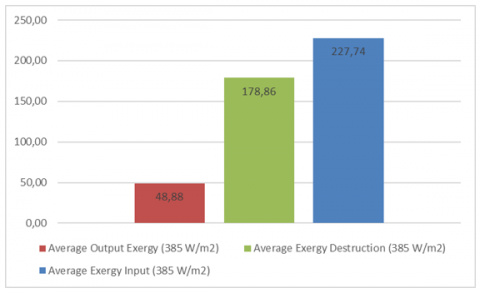Figure 2. The average of input, output and destruction exergy in 385 W/m2

## 3.png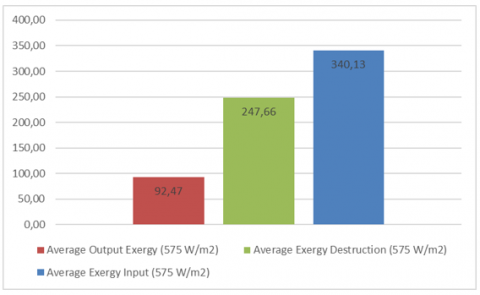Figure 3. The average of input, output and destruction exergy in 575 W/m2

## 4.png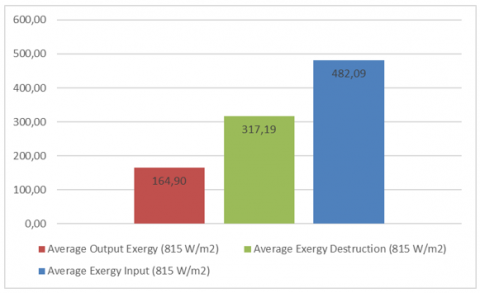Figure 4. The average of input, output and destruction exergy in 815 W/m2

## 5.png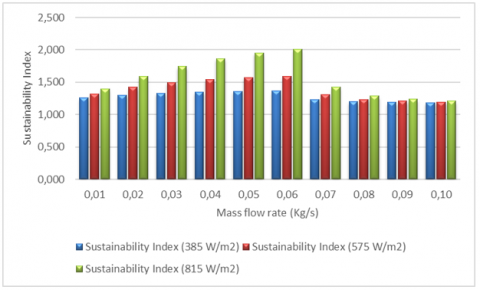Figure 5. Sustainability index with 385 W/m2, 575 W/m2, 815 W/m2

## 6.png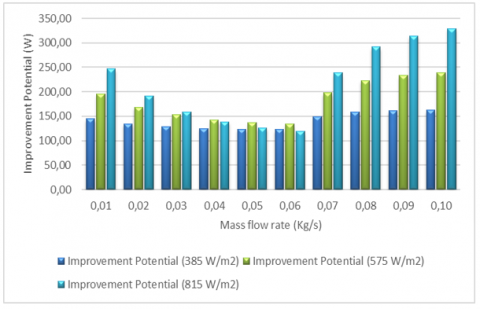Figure 6. Improvement potential with 385 W/m2, 575 W/m2, 815 W/m2

Table 4. Improvement potential and sustainability index evaluation with previous study

 Designs of collector Sustainability index Improvement potential (Watt) References, years Solar collector 1.0073 NA , 2017 Fins and non-fins NA 740-1070 , 2013 Solar for drying NA 0-17 , 2010 Solar for drying seaweed NA 0.3-630 , 2013 Solar for drying chili NA 0.135-47 , 2014 Solar for palm NA 8-455 , 2015 Solar for water NA 98-404 , 2013 Plates absorber NA NA , 2008 flat-plate solar for air heater NA NA , 2010 Nanofluid NA NA , 2016 Aluminium plate NA NA , 2017 flat transpired NA NA , 2016 Solar collector NA NA , 2016 ∇-groove 1.152–1.171 NA , 2019 flat-plate collector 1.147 – 1.174 NA , 2022 V-Absorber 1.141-1.157 119.09-329.24 Present study

Table 4 above shows that research on PVT systems with a sustainability index approach is rarely used. For the first time in 2017, a study using the sustainability index approach on solar collectors was conducted by Caliskan . The result of Caliskan's research in solar collectors was 1.0073. Subsequently, the research followed by Fudholi et al.  in 2019 on PVT system using ∇-corrugated collector model. The sustainability index value obtained was 1.152-1.171. and the sustainability index with flat-plate collector in the recent year of 2022 was 1.147-1.174 as shown in Table 2. Furthermore, the results obtained in this study using a mathematical approach to V-Absorber model are 1.141-1.157. Therefore, the result of the V-Absorber indicates conformity with previous research. Likewise, the improvement potential value obtained is very close to the results of previous researchers.

4. Conclusions

A mathematical model approach has been carried out on a PVT system using V-Absorber. The flow of air variable is from 0.01 kg/s to 0.10 kg/s, and the three variables of sunlight intensity are 385 W/m2, 575 W/m2 and 815 W/m2. The analysis result of the mathematical model shows that the effect of flow air and sunlight intensity on the system affects the input exergy, output exergy and destruction exergy in the system. The sustainability index (SI) value using V-Absorber increase the SI value in the system. The highest SI value is 2.012 at sunlight intensity of 815 W/m2 with flow air of 0.06 kg/s. For the improvement potential (IP), the highest IP value is 329.24 Watt at sunlight intensity of 815 W/m2 with flow air of 0.10 kg/s. For all sunlight intensity, the maximum SI value position is 0.06 kg/s., and the maximum IP value position is 0.10 kg/s.

Nomenclature
 T Temperature (K) A Area of PVT (m2) h heat transfer coefficient (W/m2 K) L length (m) Ub bottom loss coefficient (W/m2 K) Ut Top loss coefficient (W/m2 K) I Sunlight intensity (W/m2) $\dot{m}$ Flow air (kg/s) C specific heat of air (J/kg.K) W width of collector (m) H height of collector (m) Nu Nusselt number Re Reynolds number Pr Prandtl number Subscripts and Greek symbols c photovoltaic v V-absorber r radiative 1 The first flow 2 The second flow i Inlet of air o Outlet of air η efficiency ϕ angle between v-groove α absorption coefficient τ Transmission coefficient $a$ ambient s sky w wind
References

 Ge, Z., Wang, H., Wang, H., Zhang, S., Guan, X. (2014). Exergy analysis of flat plate solar collectors. Entropy, 16(5): 2549-2567. https://doi.org/10.3390/e16052549

 Faizal, M.S.R.M.S., Saidur, R., Mekhilef, S., Hepbasli, A., Mahbubul, I.M. (2015). Energy, economic, and environmental analysis of a flat-plate solar collector operated with SiO2 nanofluid. Clean Technologies and Environmental Policy, 17: 1457-1473. https://doi.org/10.1007/s10098-014-0870-0

 Zohri, M., Nurato, N., Bakti, L.D., Fudholi, A. (2018). Exergy assessment of photovoltaic thermal with v-groove collector using theoretical study. TELKOMNIKA (Telecommunication Computing Electronics and Control), 16(2): 550-557. https://doi.org/10.12928/TELKOMNIKA.v16i2.8433

 Caliskan, H. (2017). Energy, exergy, environmental, enviroeconomic, exergoenvironmental (EXEN) and exergoenviroeconomic (EXENEC) analyses of solar collectors. Renewable and Sustainable Energy Reviews, 69: 488-492. https://doi.org/10.1016/j.rser.2016.11.203

 Chow, T.T., He, W., Chan, A.L.S., Fong, K.F., Lin, Z., Ji, J. (2008). Computer modeling and experimental validation of a building-integrated photovoltaic and water heating system. Applied Thermal Engineering, 28(11-12): 1356-1364. https://doi.org/10.1016/j.applthermaleng.2007.10.007

 Hazi, A., Hazi, G., Grigore, R., Vernica, S. (2014). Opportunity to use PVT systems for water heating in industry. Applied Thermal Engineering, 63(1): 151-157. https://doi.org/10.1016/j.applthermaleng.2013.11.010

 Das, D., Kalita, P., Roy, O. (2018). Flat plate hybrid photovoltaic-thermal (PV/T) system: A review on design and development. Renewable and Sustainable Energy Reviews, 84: 111-130. https://doi.org/10.1016/j.rser.2018.01.002

 Al-Waeli, A.H., Sopian, K., Kazem, H.A., Chaichan, M.T. (2017). Photovoltaic/Thermal (PV/T) systems: Status and future prospects. Renewable and Sustainable Energy Reviews, 77: 109-130. https://doi.org/10.1016/j.rser.2017.03.126

 Wu, J., Zhang, X., Shen, J., Wu, Y., Connelly, K., Yang, T., Wang, H. (2017). A review of thermal absorbers and their integration methods for the combined solar photovoltaic/thermal (PV/T) modules. Renewable and Sustainable Energy Reviews, 75: 839-854. https://doi.org/10.1016/j.rser.2016.11.063

 Good, C. (2016). Environmental impact assessments of hybrid photovoltaic–thermal (PV/T) systems–A review. Renewable and Sustainable Energy Reviews, 55: 234-239. https://doi.org/10.1016/j.rser.2015.10.156

 Chow, T.T. (2010). A review on photovoltaic/thermal hybrid solar technology. Applied Energy, 87(2): 365-379. https://doi.org/10.1016/j.apenergy.2009.06.037

 Zondag, H.A. (2008). Flat-plate PV-Thermal collectors and systems: A review. Renewable and Sustainable Energy Reviews, 12(4): 891-959. https://doi.org/10.1016/j.rser.2005.12.012

 Ibrahim, A., Othman, M.Y., Ruslan, M.H., Mat, S., Sopian, K. (2011). Recent advances in flat plate photovoltaic/thermal (PV/T) solar collectors. Renewable and Sustainable Energy Reviews, 15(1): 352-365. https://doi.org/10.1016/j.rser.2010.09.024

 Hamid, S.A., Othman, M.Y., Sopian, K., Zaidi, S.H. (2014). An overview of photovoltaic thermal combination (PV/T combi) technology. Renewable and Sustainable Energy Reviews, 38: 212-222. https://doi.org/10.1016/j.rser.2014.05.083

 Kumar, R., Rosen, M.A. (2011). A critical review of photovoltaic–thermal solar collectors for air heating. Applied Energy, 88(11): 3603-3614. https://doi.org/10.1016/j.apenergy.2011.04.044

 Dincer, I. (2011). Exergy as a potential tool for sustainable drying systems. Sustainable Cities and Society, 1(2): 91-96. https://doi.org/10.1016/j.scs.2011.04.001

 Fudholi, A., Sopian, K. (2018). R&D of photovoltaic thermal (PVT) systems: An overview. International Journal of Power Electronics and Drive Systems, 9(2): 803. https://doi.org/10.11591/ijpeds.v9.i2.pp803-810

 Kalogirou, S.A., Karellas, S., Badescu, V., Braimakis, K. (2016). Exergy analysis on solar thermal systems: A better understanding of their sustainability. Renewable Energy, 85: 1328-1333. https://doi.org/10.1016/j.renene.2015.05.037

 Fudholi, A., Sopian, K., Ruslan, M.H., Othman, M.Y. (2013). Performance and cost benefits analysis of double-pass solar collector with and without fins. Energy Conversion and Management, 76: 8-19. https://doi.org/10.1016/j.enconman.2013.07.015

 Fudholi, A., Sopian, K., Othman, M.Y., Ruslan, M.H., Bakhtyar, B. (2013). Energy analysis and improvement potential of finned double-pass solar collector. Energy Conversion and Management, 75: 234-240. https://doi.org/10.1016/j.enconman.2013.06.021

 Layek, A., Saini, J.S., Solanki, S.C. (2007). Second law optimization of a solar air heater having chamfered rib-groove roughness on absorber plate. Renewable Energy, 32(12): 1967-1980. https://doi.org/10.1016/j.renene.2006.11.005

 Benli, H. (2013). Experimentally derived efficiency and exergy analysis of a new solar air heater having different surface shapes. Renewable Energy, 50: 58-67. https://doi.org/10.1016/j.renene.2012.06.022

 Hedayatizadeh, M., Ajabshirchi, Y., Sarhaddi, F., Farahat, S., Safavinejad, A., Chaji, H. (2012). Analysis of exergy and parametric study of a v-corrugated solar air heater. Heat and Mass Transfer, 48: 1089-1101. https://doi.org/10.1007/s00231-011-0957-7

 Lari, M.O., Sahin, A.Z. (2017). Design, performance and economic analysis of a nanofluid-based photovoltaic/thermal system for residential applications. Energy Conversion and Management, 149: 467-484. https://doi.org/10.1016/j.enconman.2017.07.045

 Khanjari, Y., Pourfayaz, F., Kasaeian, A.B. (2016). Numerical investigation on using of nanofluid in a water-cooled photovoltaic thermal system. Energy Conversion and Management, 122: 263-278. https://doi.org/10.1016/j.enconman.2016.05.083

 Salem, M.R., Ali, R.K., Elshazly, K.M. (2017). Experimental investigation of the performance of a hybrid photovoltaic/thermal solar system using aluminium cooling plate with straight and helical channels. Solar Energy, 157: 147-156. https://doi.org/10.1016/j.solener.2017.08.019

 Tripathi, R., Tiwari, G.N., Dwivedi, V.K. (2016). Overall energy, exergy and carbon credit analysis of N partially covered photovoltaic thermal (PVT) concentrating collector connected in series. Solar Energy, 136: 260-267. https://doi.org/10.1016/j.solener.2016.07.002

 Tiwari, G.N., Yadav, J.K., Singh, D.B., Al-Helal, I.M., Abdel-Ghany, A.M. (2015). Exergoeconomic and enviroeconomic analyses of partially covered photovoltaic flat plate collector active solar distillation system. Desalination, 367: 186-196. https://doi.org/10.1016/j.desal.2015.04.010

 Jahromi, S.N., Vadiee, A., Yaghoubi, M. (2015). Exergy and economic evaluation of a commercially available PV/T collector for different climates in Iran. Energy Procedia, 75: 444-456. https://doi.org/10.1016/j.egypro.2015.07.416

 Rajoria, C.S., Agrawal, S., Tiwari, G.N., Chaursia, G.S. (2015). Exergetic and enviroeconomic analysis of semitransparent PVT array based on optimum air flow configuration and its comparative study. Solar Energy, 122: 1138-1145. https://doi.org/10.1016/j.solener.2015.10.020

 Kamthania, D., Tiwari, G.N. (2014). Energy metrics analysis of semi-transparent hybrid PVT double pass facade considering various silicon and non-silicon based PV module Hyphen is accepted. Solar Energy, 100: 124-140. https://doi.org/10.1016/j.solener.2013.11.015

 Karim, M.A., Hawlader, M.N.A. (2006). Performance investigation of flat plate, v-corrugated and finned air collectors. Energy, 31(4): 452-470. https://doi.org/10.1016/j.energy.2005.03.007

 Zohri, M., Fudholi, A., Ruslan, M.H., Sopian, K. (2017). Mathematical modeling of photovoltaic thermal PV/T system with v-groove collector. In AIP Conference Proceedings, 1862(1): 030063. https://doi.org/10.1063/1.4991167

 Gupta, M.K., Kaushik, S.C. (2008). Exergetic performance evaluation and parametric studies of solar air heater. Energy, 33(11): 1691-1702. https://doi.org/10.1016/j.energy.2008.05.010

 Akpinar, E.K. (2010). Drying of mint leaves in a solar dryer and under open sun: modelling, performance analyses. Energy Conversion and Management, 51(12): 2407-2418. https://doi.org/10.1016/j.enconman.2010.05.005

 Fudholi, A., Sopian, K., Othman, M.Y., Ruslan, M.H. (2014). Energy and exergy analyses of solar drying system of red seaweed. Energy and Buildings, 68: 121-129. https://doi.org/10.1016/j.enbuild.2013.07.072

 Fudholi, A., Sopian, K., Yazdi, M.H., Ruslan, M.H., Gabbasa, M., Kazem, H.A. (2014). Performance analysis of solar drying system for red chili. Solar Energy, 99: 47-54. https://doi.org/10.1016/j.solener.2013.10.019

 Fudholi, A., Sopian, K., Alghoul, M.A., Ruslan, M.H., Othman, M.Y. (2015). Performances and improvement potential of solar drying system for palm oil fronds. Renewable Energy, 78: 561-565. https://doi.org/10.1016/j.renene.2015.01.050

 Ibrahim, A., Fudholi, A., Sopian, K., Othman, M.Y., Ruslan, M.H. (2014). Efficiencies and improvement potential of building integrated photovoltaic thermal (BIPVT) system. Energy Conversion and Management, 77: 527-534. https://doi.org/10.1016/j.enconman.2013.10.033

 Esen, H. (2008). Experimental energy and exergy analysis of a double-flow solar air heater having different obstacles on absorber plates. Building and Environment, 43(6): 1046-1054. https://doi.org/10.1016/j.buildenv.2007.02.016

 Akpinar, E.K., Koçyiğit, F. (2010). Energy and exergy analysis of a new flat-plate solar air heater having different obstacles on absorber plates. Applied Energy, 87(11): 3438-3450. https://doi.org/10.1016/j.apenergy.2010.05.017

 Gholampour, M., Ameri, M. (2016). Energy and exergy analyses of Photovoltaic/Thermal flat transpired collectors: Experimental and theoretical study. Applied Energy, 164: 837-856. https://doi.org/10.1016/j.apenergy.2015.12.042

 Hazami, M., Riahi, A., Mehdaoui, F., Nouicer, O., Farhat, A. (2016). Energetic and exergetic performances analysis of a PV/T (photovoltaic thermal) solar system tested and simulated under to Tunisian (North Africa) climatic conditions. Energy, 107: 78-94. https://doi.org/10.1016/j.energy.2016.03.134

 Fudholi, A., Zohri, M., Rukman, N.S.B., Nazri, N.S., Mustapha, M., Yen, C.H.,  Sopian, K. (2019). Exergy and sustainability index of photovoltaic thermal (PVT) air collector: A theoretical and experimental study. Renewable and Sustainable Energy Reviews, 100: 44-51. https://doi.org/10.1016/j.rser.2018.10.019

 Zohri, M., Ramadhan, W., Fudholi, A. (2022). Improvement potential and sustainability index of photovoltaic thermal solar air collector. International Journal of Heat and Technology, 40(3): 743-748. https://doi.org/10.18280/ijht.400312